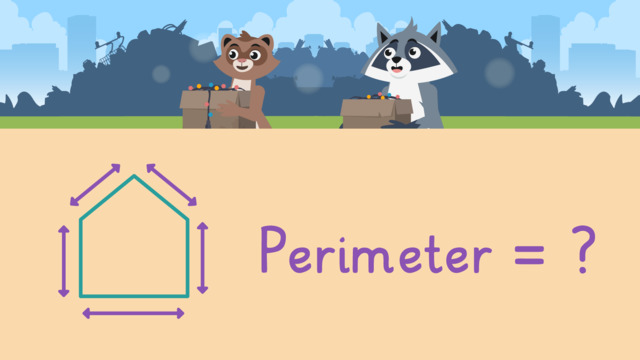# Finding Perimeter with Given Side LengthsRate this video

Ø 5.0 / 1 ratings

The authorTeam Digital
Finding Perimeter with Given Side Lengths
CCSS.MATH.CONTENT.3.MD.D.8

## Finding Perimeter with Given Side Lengths Exercise

Would you like to practice what you’ve just learned? Practice problems for this video Finding Perimeter with Given Side Lengths help you practice and recap your knowledge.
• ### What is the perimeter of the yard?

Hints

Remember, the perimeter is the distance around the shape. It is the total length of all the shape's sides.

As an example, this is how you would find the perimeter for the rectangle below.

Solution

The perimeter of a shape is the distance around the shape.
To find the perimeter, we must find the sum of the shape's sides by adding.

10 + 15 + 10 + 15 = 50
The perimeter of the yard is 50 yards.

• ### How do you find the perimeter of a shape?

Hints

Looking at the picture of the rectangle, what step is first to find the perimeter?

To find the perimeter, you must add the length of all sides of the shape.

Remember- it is important to include the measurement units!

Solution

Perimeter is the distance around an object. To find the perimeter you must:

1. Identify the side lengths and units of the figure.
3. Add by calculating the sum of the sides.
4. Rewrite the sum with the correct units next to the perimeter.
• ### What is the perimeter of each shape?

Hints

The perimeter of a shape is the distance around the shape. It is the total length of the shape's sides. To find the perimeter, find the total length of the shape's sides.

As an example, this is how you would find the perimeter for the shape below.

Solution

Perimeter is the distance around a shape. To find the perimeter, find the total length of the shape's sides.

Rectangle: 7 + 20 + 7 + 20 = 54
The perimeter of the rectangle is 54 inches.

Triangle: 15 + 15 + 15 = 45
The perimeter of the triangle is 45 inches.

Square: 12 + 12 + 12 + 12 = 48
The perimeter of the square is 48 inches.

Rhombus: 13 + 13 + 13 + 13 = 52
The perimeter of the rhombus is 52 inches.

• ### What is the perimeter of each window?

Hints

The perimeter of a shape is the distance around the shape.

Add the lengths of the sides to find the total distance around the shape.

As an example, this is how you would find the perimeter for the shape below.

Solution

The perimeter of a shape is the distance around the shape. To find the perimeter find the total length of the shape's sides.

Window 1:
16 + 12 + 14 + 18 = 60
The perimeter of window 1 is 60 inches.

Window 2:
14 + 28 + 14 + 28 = 84
The perimeter of window 2 is 84 inches.

Window 3:
14 + 22 + 18 + 28 = 82
The perimeter of window 3 is 82 inches.

• ### What is the perimeter of the pentagon?

Hints

The perimeter of a shape is the distance around the shape. It is the total length of the shape's sides.

To calculate the perimeter, you must find the sum the shape's sides. As an example, this is how you would find the perimeter for the shape below.

Solution

The perimeter of a shape is the distance around the shape. It is the total length of the shape's sides.

To find the perimeter, add the lengths of each side of the pentagon.

3 + 3 + 3 + 3 + 3 = 15

The perimeter of the pentagon is 15 centimeters.

• ### Which shapes have a perimeter of 24 feet?

Hints

More than one shape has a perimeter of 24 feet.

Perimeter is the distance around a shape. As an example, this is how you would find the perimeter for the rectangle below.

Solution

The perimeter of a shape is the distance around the shape. It is the total length of the shape's sides.

To find the perimeter:

1. Identify the side lengths and labels.
2. Add to find the sum, or total length, of the perimeter of the shape.

Square:
6 + 6 + 6 + 6 = 24
The perimeter of the square is 24 feet.

Rectangle:
3 + 9 + 3 + 9 = 24
The perimeter of the rectangle is 24 feet.

Pentagon:
5 + 5 + 5 + 5 + 5 = 25
The perimeter of the pentagon is NOT 24 feet.

Triangle:
8 + 8 + 8 = 24
The perimeter of the triangle is 24 feet.